# Roxville

The temperature in Roxville decreased 15 degree in five hours. What was the average decrease in temperature per hour? Write you answer as an integer.

a =  3 °C/h

### Step-by-step explanation: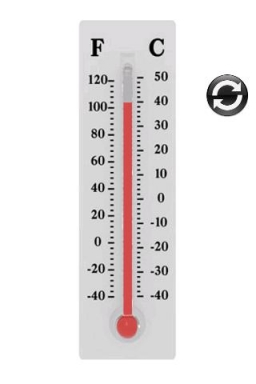Did you find an error or inaccuracy? Feel free to write us. Thank you!Tips to related online calculators
Looking for help with calculating arithmetic mean?
Looking for a statistical calculator?
Do you want to convert time units like minutes to seconds?

#### You need to know the following knowledge to solve this word math problem:

We encourage you to watch this tutorial video on this math problem:

## Related math problems and questions:

• The temperature 14The temperature is 12°C lower than 4 hours ago. a. What is the average temperature change per hour? b. Write an integer equation for the situation.
• Difference in temperaturesThe temperature in the number line show minus 15 degree celsius and 8 degree celsius. What is the difference between the temperatures?
• NoontimeIn the morning temperature was 110°F. By noontime, it has gone up by 15°F. What was the noon temperature? (Give your answer in °F)
• Temperature changeThe mean temperature change is -3.2°F per day for five days. What is the total change over the five day period?
• Equal temperature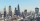The temperatures of the two cities were measured at the same time. The temperature in city A was 60 degrees And rose at a constant rate of 2 degrees per hour. The temperature in city B was 40° and rose at a constant rate of 10° per hour Enter the time in
• If the 3If the temperature of a city drops 2° everyday and increases by 3° on every 5 days, how long does it take to drop to -15° from temperature +5°?
• The temperature 3The temperature is -12 degrees Fahrenheit. During the following 5 hours, the temperature decrease by 23 degrees Fahrenheit. What is the weather at 11 pm?
• The temperature 11The temperature on Sunday was 4°C. The temperature dropped 8°C on Monday and twice as much on Tuesday. What is the temperature on Tuesday?
• The temperature 10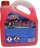The temperature in a freezer is -15°C, and it increases by 3°C.
• The temperature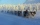The temperature is 0 degrees. It drops 5 degrees after 1 hour and then drops 3 degrees more the next hour. What is the temperature after 6 hours?
• Temperature variationsToday's temperature was 80 degrees, and then the temperature dropped 10 degrees. Then it dropped 15 degrees again, then the next day, the temperature went up 2 degrees. What would the temperature be?
• Change per hour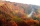On Sunday, the temperature was 63 degrees at 2 pm. The temperature was then 72 degrees at 5 pm. What is the rate of change per hour?
• 15 numberWhat number is smaller (greater) by 15 than its half?
• Temperature change 2The outside temperature changed -14F over 4 hours. If the temperature changed the same amount each hour, what was the change in temperature each hour?
• A cold front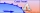At 1:00 PM, the temperature was at 46 degrees. Then, a cold front moved in and decreased the temperature 12 degrees per hour. The temperature at 6:00 PM = _____ degrees.
• Temperature differenceLibya's highest temperature was recorded at 58 degrees Celsius, and the lowest was recorded at -88 degrees Celsius. What is the temperature difference?
• Outdoor temperatureThe outdoor temperature was 8 degrees at midnight. The temperature declined 5 degrees during each of the next 3 hours. What was the temperature at 3am?